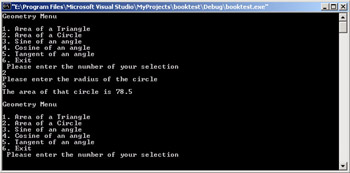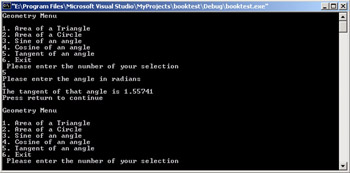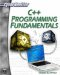# Creating and Using a Class

Another important item to keep in mind is that a class is a template for creating objects. When you create a class you are essentially creating a new data type, one that is complex and has its own functions or methods. You cannot simply start using the class, you must first create an instance of it. This is not different from any other data type. You cannot, for example, write the following.

`int = 7;`

The int is a data type, and not an actual variable. You must first create a specific int before you can assign values to it.

`int j = 7;`

The same is true of your classes. The process of creating an instance of a class has several names, all of which mean the same thing. You will hear this referred to as instantiating a class, creating an instance of a class, or creating an object from a class. All of these simply mean that you are creating a specific variable of the type of your class.

Let’s examine another example of a class; this one will probably be more useful than our first example.

### Example 10.2

Step 1: Enter the following code into your favorite text editor and save it as 010-02.h.

`#include <cmath> class myfirstclass {       float answer;       double danswer; public:  float areaofcircle(float);  float areaoftriangle(float, float);  double sineofangle(double);  double cosineofangle(double);  double tangentofangle(double); }; float myfirstclass::areaofcircle(float radius) {    answer = 3.14f * (pow(radius,2));        return answer; } float myfirstclass::areaoftriangle(float b, h) {   answer = .5f * (b * h);       return answer; } double myfirstclass::sineofangle(double angle) {   danswer = sin(angle);       return danswer; } double myfirstclass::cosineofangle(double angle) {    danswer = cos(angle);        return danswer; } double myfirstclass::tangentofangle(double angle) {   danswer = tan(angle);       return danswer; }`

Step 2: Enter this code into your favorite text editor and save it as 10-2.cpp.

`#include "010-02.h" #include <iostream> using namespace std;   // function prototype void menu(); // an instance of our class myfirstclass myfirstobject; int main ()  {  menu();      return 0;    }    void menu()    { double danswer, angle;      float radius, base, height, area;  int imenu;      cout << "Geometry Menu \n \n";      cout << "1. Area of a Triangle \n";  cout << "2. Area of a Circle \n";      cout << "3. Sine of an angle \n";      cout << "4. Cosine of an angle \n";      cout << "5. Tangent of an angle \n";      cout << "6. Exit \n";      cout << "Please enter the number of your selection   \n";      cin >> imenu;      switch(imenu)      {       case 1:    cout << "Please enter the base of the triangle \n";        cin >> base;        cout << "Please enter the height of the triangle  \n";        cin >> height;        area =myfirstobject.areaoftriangle(base, height);        cout << "The area of that triangle is " << area <<   "\n";        cout << " \n";        menu();        break;       case 2:    cout << "Please enter the radius of the circle\n";        cin >> radius;    area = myfirstobject.areaofcircle(radius);        cout << "The area of that circle is " << area <<  "\n";        cout << " \n";        menu();        break;       case 3:    cout << "Please enter the angle in radians \n";        cin >> angle;        danswer =myfirstobject.sineofangle(angle);        cout << "The sine of that angle is " << danswer <<  "\n";        cout << " \n";        menu();        break;       case 4:    cout << "Please enter the angle in radians \n";        cin >> angle;        danswer = myfirstobject.cosineofangle(angle);        cout << "The cosine of that angle is " << danswer <<  "\n";        cout << " \n";        menu();        break;       case 5:    cout << "Please enter the angle in radians \n";        cin >> angle;        danswer =myfirstobject.tangentofangle(angle);    cout << "The tangent of that angle is " << danswer   << "\n";        cout << "Press return to continue \n";        cout << " \n";        menu();       case 6:    return; // simply go back to main        break;       default:      cout << "Please make a valid choice \n";      menu(); // call this function again to display the     // menu again      }// end of switch }`

Step 3: Compile the code and run it. You will see a series of images much like those depicted in Figures 10.2 and 10.3.Figure 10.2: Geometry class.Figure 10.3: Geometry class 2.

Clearly this particular program is a bit longer and more complex than most of the examples you have seen previously in this book. However, don’t let that disturb you. The principles are the same as the simpler examples. We have taken some basic geometric and trigonometric functions and placed them in a class. That class is defined inside a header file. Instances of that class can be created inside any source code file where you may require the use of those functions. This previous example illustrates several points. To begin with, you see an example of the math functions found in math.h, as you had seen once before in a previous chapter. Also in this example we saw the use of a class, the creation and use of a header file, and finally the use of a menu function. This example is essentially the culmination of this book to this point and is of practical value, particularly to any readers currently engaged in the study of elementary geometry or trigonometry.C++ Programming Fundamentals (Cyberrookies)
ISBN: 1584502371
EAN: 2147483647
Year: 2005
Pages: 197
Authors: Chuck Easttom